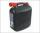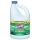# The pool

The pool contains 220 m3 of water. The pool can be emptied either:
a) 10 hours of pipe B and 8 hours of pipe A, or
b) 10 hours of pipe A and 7 hours of pipe B.

How many cubic meters of water will flow in 1 hour from pipe A and how many from pipe B?

Result

A =  15
B =  10

#### Solution:

10*B + 8*A = 220
10*A + 7*B = 220

8A+10B = 220
10A+7B = 220

A = 15
B = 10

Calculated by our linear equations calculator.

Leave us a comment of example and its solution (i.e. if it is still somewhat unclear...):

Showing 0 comments:Be the first to comment!#### To solve this example are needed these knowledge from mathematics:

Looking for help with calculating harmonic mean? Looking for a statistical calculator? Do you have a system of equations and looking for calculator system of linear equations? Tip: Our volume units converter will help you with converion of volume units.

## Next similar examples:

1. BottlesThe must is sold in 5-liter and 2-liter bottles. Mr Kucera bought a total of 216 liters in 60 bottles. How many liters did Mr. Kucera buy in five-liter bottles?
2. Gasoline canisters35 liters of gasoline is to be divided into 4 canisters so that in the third canister will have 5 liters less than in the first canister, the fourth canister 10 liters more than the third canister and the second canister half of that in the first canist
3. Three workshopsThere are 2743 people working in three workshops. In the second workshop works 140 people more than in the first and in third works 4.2 times more than the second one. How many people work in each workshop?
4. Gasoline35 l of gasoline is to be divided into four canisters so that in the third canister was 5 l less than the first canister, the fourth canister 10 l more than in the third canister and the second canister half what in the first canister. How many liters of.
5. Theorem proveWe want to prove the sentense: If the natural number n is divisible by six, then n is divisible by three. From what assumption we started?
6. LegsCancer has 5 pairs of legs. The insect has 6 legs. 60 animals have a total of 500 legs. How much more are cancers than insects?
7. Linsys2Solve two equations with two unknowns: 400x+120y=147.2 350x+200y=144
8. Three unknownsSolve the system of linear equations with three unknowns: A + B + C = 14 B - A - C = 4 2A - B + C = 0
9. Elimination methodSolve system of linear equations by elimination method: 5/2x + 3/5y= 4/15 1/2x + 2/5y= 2/15
10. Home cleaningMr. Smith is cleaning up a big mess at home. In the closet, he finds a solution that is 5% bleach, and another stronger solution that is 20% bleach. For this particular job, he needs 600mL of 15% bleach. How much of each type (to the nearest mL) should he.
11. Alcohol mixingHow much 55% alcohol we must pour into 1500 g 80% alcohol to form a 60% alcohol? How much 60% alcohol created?
12. FamilyFamily has 4 children. Ondra is 3 years older than Matthew and Karlos 5 years older than the youngest Jane. We know that they are together 30 years and 3 years ago they were together 19 years. Determine how old the children are.
13. Boys and money270 USD boys divided so that Peter got three times more than Paul and Ivan has 120 USD more than than Paul. How much each received?
14. Father 7Father is 6 times older than his son. After 4 years, the father will only be 4 times older. What are their present ages?
15. 925 USDFour classmates saved an annual total 925 USD. The second save twice as the first, third 35 USD more than the second and fourth 10 USD less than the first. How USD save each of them?
16. Two equationsSolve equations (use adding and subtracting of linear equations): -4x+11y=5 6x-11y=-5
17. Three friendsDanica, Lenka and Dalibor have altogether 96 kg. Lenka weighs 75% more than Dalibor and Danica weighs 6 kg more than Dalibor. Determine the weight of Danice, Lenka and Dalibor.